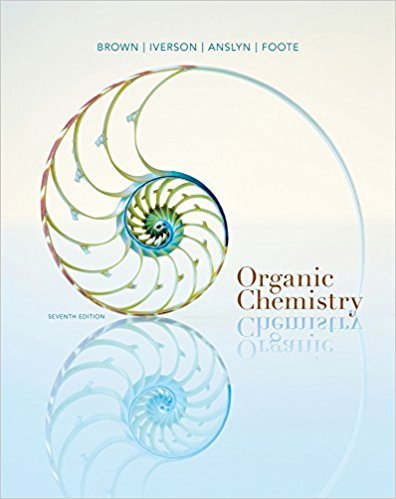×
Get Full Access to Organic Chemistry - 7 Edition - Chapter 14 - Problem 14.11
Get Full Access to Organic Chemistry - 7 Edition - Chapter 14 - Problem 14.11

×

# Determine the probability of the following in a natural sample of ethane.(a) One carbonISBN: 9781133952848 483

## Solution for problem 14.11 Chapter 14

Organic Chemistry | 7th Edition

• Textbook Solutions
• 2901 Step-by-step solutions solved by professors and subject experts
• Get 24/7 help from StudySoup virtual teaching assistantsOrganic Chemistry | 7th Edition

4 5 1 244 Reviews
25
3
Problem 14.11

Determine the probability of the following in a natural sample of ethane.(a) One carbon in an ethane molecule is 13C.(b) Both carbons in an ethane molecule are 13C.(c) Two hydrogens in an ethane molecule are replaced by deuterium atoms.

Step-by-Step Solution:
Step 1 of 3

ORGO: Chapter 1 Notes Hannah Malcomson 1.1-  Organic compounds are difficult to isolate and decompose more easily than inorganic compounds. o Scientists originally thought that organic molecules contained a ‘special vital force’- vitalism. o Vitalism was disproved, current definition of an organic molecule was created  Organic molecule: a compound containing carbon atoms o Inorganic molecule: a compound with no carbon molecules 1.2-  Constitutional Isomers- compounds with the same molecular formula, but have a different structure (atoms are connected in different orders)  Each element will form a (generally) predictable number of bonds o Carbon- 4 electrons (e-), 4 bonds. Tetravalent o

Step 2 of 3

Step 3 of 3

##### ISBN: 9781133952848

The answer to “Determine the probability of the following in a natural sample of ethane.(a) One carbon in an ethane molecule is 13C.(b) Both carbons in an ethane molecule are 13C.(c) Two hydrogens in an ethane molecule are replaced by deuterium atoms.” is broken down into a number of easy to follow steps, and 39 words. This full solution covers the following key subjects: . This expansive textbook survival guide covers 24 chapters, and 1131 solutions. Since the solution to 14.11 from 14 chapter was answered, more than 229 students have viewed the full step-by-step answer. Organic Chemistry was written by and is associated to the ISBN: 9781133952848. The full step-by-step solution to problem: 14.11 from chapter: 14 was answered by , our top Chemistry solution expert on 03/16/18, 05:02PM. This textbook survival guide was created for the textbook: Organic Chemistry, edition: 7.

Unlock Textbook Solution